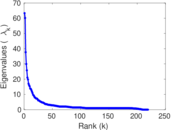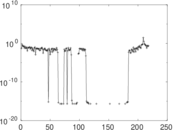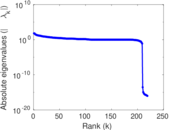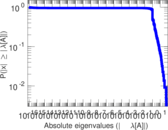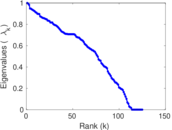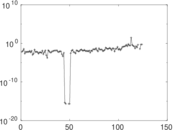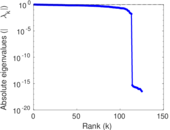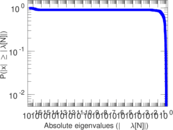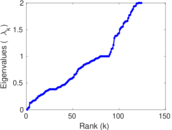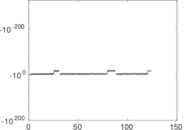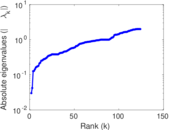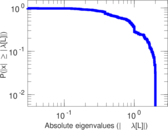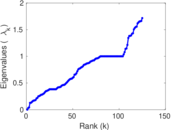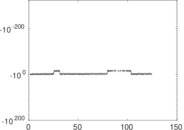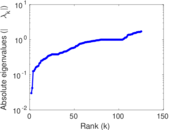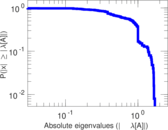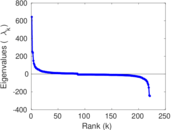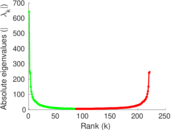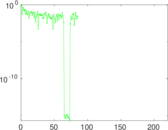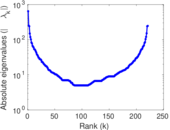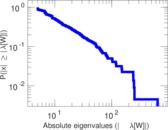# Wikipedia edits (ng)

This is the bipartite edit network of the Ndonga Wikipedia. It contains users and pages from the Ndonga Wikipedia, connected by edit events. Each edge represents an edit. The dataset includes the timestamp of each edit.

 Code `ng` Internal name `edit-ngwiki` Name Wikipedia edits (ng) Data source http://dumps.wikimedia.org/ AvailabilityDataset is available for download Consistency checkDataset passed all tests Category Authorship network Dataset timestamp 2017-10-20 Node meaning User, article Edge meaning Edit Network formatBipartite, undirected Edge typeUnweighted, multiple edges Temporal dataEdges are annotated with timestamps

## Statistics

 Size n = 663 Left size n1 = 221 Right size n2 = 442 Volume m = 1,897 Unique edge count m̿ = 875 Wedge count s = 9,244 Claw count z = 82,239 Cross count x = 703,240 Square count q = 5,613 4-Tour count T4 = 84,066 Maximum degree dmax = 160 Maximum left degree d1max = 160 Maximum right degree d2max = 118 Average degree d = 5.722 47 Average left degree d1 = 8.583 71 Average right degree d2 = 4.291 86 Fill p = 0.008 957 64 Average edge multiplicity m̃ = 2.168 00 Size of LCC N = 403 Diameter δ = 12 50-Percentile effective diameter δ0.5 = 4.514 54 90-Percentile effective diameter δ0.9 = 6.493 66 Median distance δM = 5 Mean distance δm = 4.935 72 Gini coefficient G = 0.710 330 Balanced inequality ratio P = 0.208 751 Left balanced inequality ratio P1 = 0.180 812 Right balanced inequality ratio P2 = 0.228 255 Relative edge distribution entropy Her = 0.888 245 Power law exponent γ = 3.143 37 Tail power law exponent γt = 2.131 00 Tail power law exponent with p γ3 = 2.131 00 p-value p = 0.028 000 0 Left tail power law exponent with p γ3,1 = 1.851 00 Left p-value p1 = 0.296 000 Right tail power law exponent with p γ3,2 = 2.391 00 Right p-value p2 = 0.000 00 Degree assortativity ρ = −0.132 024 Degree assortativity p-value pρ = 8.969 63 × 10−5 Spectral norm α = 63.296 7 Algebraic connectivity a = 0.030 004 1 Spectral separation |λ1[A] / λ2[A]| = 1.052 46 Controllability C = 231 Relative controllability Cr = 0.355 932

## Plots

### Fruchterman–Reingold graph drawing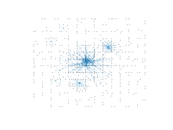### Degree distribution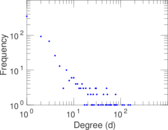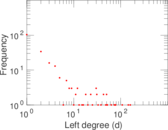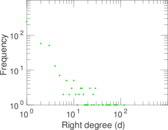### Cumulative degree distribution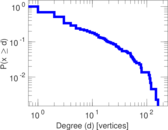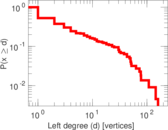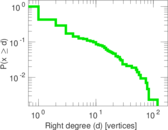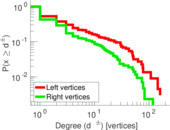### Lorenz curve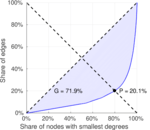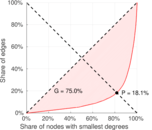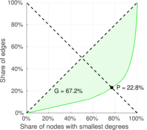### Spectral distribution of the adjacency matrix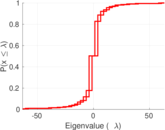### Spectral distribution of the normalized adjacency matrix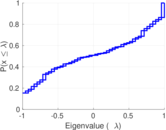### Spectral distribution of the Laplacian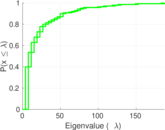### Spectral graph drawing based on the adjacency matrix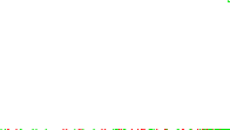### Spectral graph drawing based on the Laplacian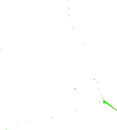### Spectral graph drawing based on the normalized adjacency matrix### Degree assortativity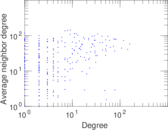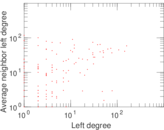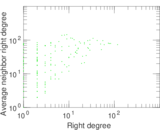### Zipf plot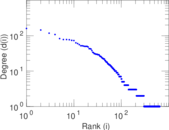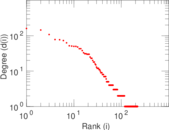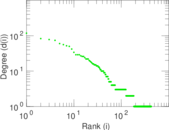### Hop distribution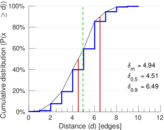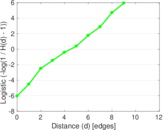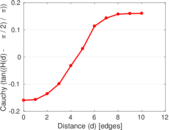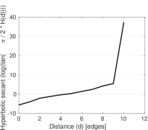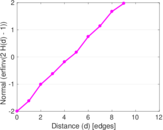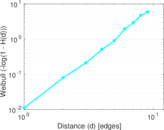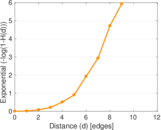### Double Laplacian graph drawing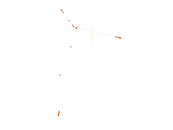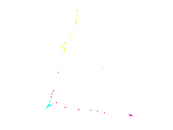### Delaunay graph drawing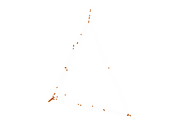### Edge weight/multiplicity distribution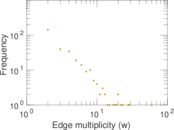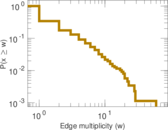### Temporal distribution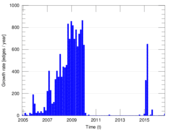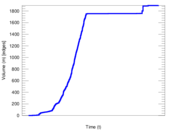### Temporal hop distribution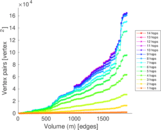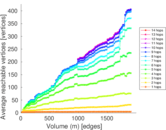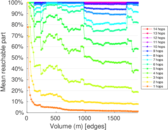### Diameter/density evolution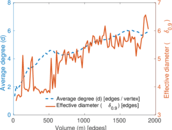### Matrix decompositions plots